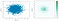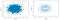# My Understanding of Exploratory Data Analysis

Exploratory data analysis (EDA), pioneered by John Tukey, set a foundation for the field of data science. The key idea of EDA is that the first and most important step in any project based on data is to look at the data. By summarizing and visualizing the data, you can gain valuable intuition and understanding of the project. Exploratory analysis should be a cornerstone of any data science project.

# Part 1: Location Estimator

Trimmed mean

• The data collected does not equally represent the different groups that we are interested in measuring. For example, because of the way an online experiment was conducted, we may not have a set of data that accurately reflects all groups in the user base. To correct that, we can give a higher weight to the values from the groups that were underrepresented.
`np.average(state['Murder.Rate'], weights=state['Population'])wquantiles.median(state['Murder.Rate'], weights=state['Population'])`
• While robust estimators (median, trimmed mean, weighted mean/median) are valid for small data sets, they do not provide added benefit for large or even moderately sized data sets.
• Statisticians and data scientists use different terms for the same thing. Statisticians use the term estimate while data scientists use the term metric for location statistics.
• location and variability are referred to as the first and second moments of a distribution. The third and fourth moments are called skewness and kurtosis. Skewness refers to whether the data is skewed to larger or smaller values; kurtosis indicates the propensity of the data to have extreme values.

# Part 2: Variability Estimator

Deviation: the difference between the observed values and the estimate of location.

`from statsmodels import robustdata = np.random.randn(30, 2)df = pd.DataFrame(data, columns=['column_1','column_2'])print(df['column_1'].std())print(robust.scale.mad(df['column_1']))`
`data = np.random.randn(30, 2)df = pd.DataFrame(data, columns=['column_1','column_2'])df.head(3)df['column_1'].quantile(0.75)-df['column_1'].quantile(0.25)`
`df['column_1'].quantile([0.05, 0.75, 0.95])`

# Part 3: Distribution Estimator

Boxplots

• top/bottom horizontal thin line in : 75th and 25th percentiles
• the dashed lines are called whiskers, and it will extend to the furthest point within 1.5 times the Interquartile Range (IQR). Any data outside of the whiskers is plotted as single points or circles (often considered as outliers).
• range: the y-coordinate range
`df['column_1'].plot.box()`
`sns.violinplot(df['column_1'], inner='quartile')`
`binned_column = pd.cut(df['column_1'], 10)print(type(binned_column))binned_column.value_counts(sort=False)`
`ax = df['column_1'].plot.hist(figsize=(6, 6))ax.set_xlabel('range value')`
`ax = df['column_1'].plot.hist(figsize=(6, 6),bins=11)df['column_1'].plot.density(ax=ax)ax.set_xlabel('range value')`
`ax=df['column_1'].plot.bar()/pie()ax.set_xlabel('')ax.set_ylabel('')`

# Part 4: Correlation

Variables X and Y (each with measured data) are said to be positively correlated if high values of X go with high values of Y, and low values of X go
with low values of Y. If high values of X go with low values of Y, and vice versa, the variables are negatively correlated. We can use `heatmap` to visualize the correlation matrix.

`print(df.corr())sns.heatmap(df.corr())`
`df.plot.scatter(x='column_1', y='column_2')`left: scatter; right: hexagonal binning
`df.plot.hexbin(x='column_1', y='column_2')`left: scatter; right: contour
• hexagonal binning and contour plot: they are used when there are a lot of data records
• heat map: it is often used to illustrate matrix. For example, correlation matrix, confusion matrix and so on

# Part 5: Contingency Table

A contingency table (also known as a cross tabulation or crosstab) is a type of table in a matrix format that displays the (multivariate) frequency distribution of the variables.

`import pandas import numpy   # creating some data a = numpy.array(["foo", "foo", "foo", "foo",                  "bar", "bar", "bar", "bar",                  "foo", "foo", "foo"],                 dtype=object)   b = numpy.array(["one", "one", "one", "two",                  "one", "one", "one", "two",                  "two", "two", "one"],                 dtype=object)   res = pandas.crosstab(a, [b], rownames=['a'], colnames=['b']) res`

# Part 6: More Variables (more than 2)

Both boxplots and voilinplots support multiple variables. For example:

`airline_stats.boxplot(by='airline', column='pct_carrier_delay')sns.violinplot(airline_stats['airline'], airline_stats['pct_carrier_delay'])`
`loans_income = pd.read_csv(LOANS_INCOME_CSV, squeeze=True)sample_data = pd.DataFrame({    'income': loans_income.sample(1000),    'type': 'Data',})sample_mean_05 = pd.DataFrame({    'income': [loans_income.sample(5).mean() for _ in range(1000)],    'type': 'Mean of 5',})sample_mean_20 = pd.DataFrame({    'income': [loans_income.sample(20).mean() for _ in range(1000)],    'type': 'Mean of 20',})results = pd.concat([sample_data, sample_mean_05, sample_mean_20]) g = sns.FacetGrid(results, col='type', col_wrap=1,                   height=2, aspect=2)g.map(plt.hist, 'income', range=[0, 200000], bins=40)g.set_axis_labels('Income', 'Count')g.set_titles('{col_name}')plt.tight_layout()plt.show()`

t-SNE

Book